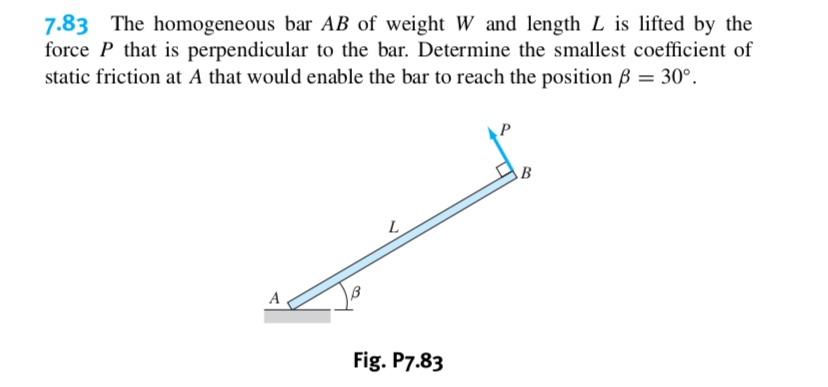# 7.83 The homogeneous bar AB of weight W and length L is lifted by theforce P that is perpendicular to the bar. Determine the smallest coefficient ofstatic friction at A that would enable the bar to reach the position B 30°ВLAFig. P7.83

Question
11 viewshelp_outlineImage Transcriptionclose7.83 The homogeneous bar AB of weight W and length L is lifted by the force P that is perpendicular to the bar. Determine the smallest coefficient of static friction at A that would enable the bar to reach the position B 30° В L A Fig. P7.83 fullscreen
check_circle

Step 1

The resultant force due to the weight of the homogeneous bar AB acts at a distance of L/2 from A and B.

Show the free body diagram of the homogeneous bar AB as in Figure (1).

Step 2

Consider counter clockwise moment is positive and clockwise moment is negative.

Determine the force P using the Equation of equilibrium;

Step 3

Consider upward force is positive.

Determine the normal force using the Equation of...

### Want to see the full answer?

See Solution

#### Want to see this answer and more?

Solutions are written by subject experts who are available 24/7. Questions are typically answered within 1 hour.*

See Solution
*Response times may vary by subject and question.
Tagged in

### Solid Mechanics